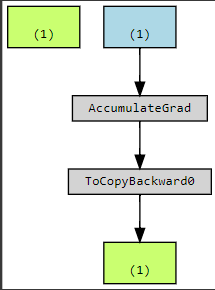Hello, I am using vision transformers in order to classify images. However, when I finished the architecture(combines viT + other moduls) I’ve found that my model is not learning so I tried to visualize the grad using make_dot and the output was as follows, can you help with any suggestion ?Or at least the relevant part

#we pass the image_filename as input, we read the image and the corresponded two filenames, each file is for a specified class 0 or 1.

#and we return the patches from this image and the target 0/1
‘’’
class PatchEmbedLeft(nn.Module):

``````#we split the image into patches and then we embedd them

def __init__(self, in_chans = 3, embed_dim = 768):

super().__init__()

self.path   ='/content/TrainingData/'

def get_coordinates(self, source,image_filename):

x = [x.split(',') for x in open(source + os.path.splitext(image_filename)+".txt").readlines()][1:]

y = [y.split(',') for y in open(source + os.path.splitext(image_filename)+".txt").readlines()][1:]

duration = [duration.split(',') for duration in open(source + os.path.splitext(image_filename)+".txt").readlines()][1:]

#map the coordinates from string to int

x = list(map(int, x))

y = list(map(int, y))

duration = list(map(int, duration))

return x,y,duration

def crop_images(self, X, Y, DURATION, image_filename):

patch_size = 32

resize = transforms.Resize([224, 224])

to_tensor = transforms.ToTensor()

self.normalize = transforms.Normalize([0.49139968, 0.48215841, 0.44653091], [0.24703223, 0.24348513, 0.26158784])

self.projection = nn.Conv2d(3, 768, kernel_size=patch_size*2, stride=patch_size*2).to(device)

cropped_patches = torch.tensor([]).to(device)

for x, y, duration in zip(X, Y, DURATION):

if duration > 500:

img = Image.open(self.path+'Images/'+image_filename).convert('RGB')

img = img.crop((x-patch_size, y-patch_size, x+patch_size, y+patch_size))

tensor = to_tensor(img)

tensor = tensor.to(device)

tensor = self.normalize(tensor)

img = tensor.unsqueeze(0)

img = self.projection(img)

cropped_patches = torch.cat((cropped_patches, img),0) #[nb_patches, embedd_dim, size,size]

return cropped_patches

def get_patches(self, image_filename):

#because each image can be classified as both 0/1 so each training step we chose randomly what class to take

#notice that for each case, the label is different, first is torch.ones and second is torch.zeros

images = []

i = random.choice([0,1])

if i ==1:

x_ASD,y_ASD,duration_ASD = self.get_coordinates(self.path+"ASD/ASD_scanpath_", image_filename)

ASD_cropped_patches = self.crop_images(x_ASD, y_ASD, duration_ASD, image_filename)

return ASD_cropped_patches, label_ASD

else:

x_ASD,y_ASD,duration_ASD = self.get_coordinates(self.path+"TD/TD_scanpath_", image_filename)

ASD_cropped_patches = self.crop_images(x_ASD, y_ASD, duration_ASD, image_filename)

return ASD_cropped_patches, label_ASD

def forward(self, image_filename):

x_ASD, label_ASD = self.get_patches(image_filename)

x_ASD = x_ASD.flatten(1)  #output size of each one: [nbr_patches, embed_dim=768]

return x_ASD, label_ASD
``````

‘’’

and the global model is the following
‘’’
class Model(nn.Module):

def init(self):

``````super().__init__()

self.vit_right = VisionTransformerRight()

self.vit_left  = VisionTransformerLeft()

self.crossAttention = CrossAttention()

self.final_block = FinalBlock(nbr_modules=1)
``````

def forward(self, image_filename):

``````output_right = self.vit_right(image_filename)

output_left, label_left = self.vit_left(image_filename)

output  = self.crossAttention(output_right, output_left)

#the class_predicted is equal to torch.zeros and will be updated based on the label returned
class_predicted = torch.zeros()

class_predicted = self.final_block(output)

return  class_predicted, label_left
``````

‘’’

may be @ptrblck could help please ?

Here you only posted the definition for `PatchEmbedLeft` and `Model` but not how `VisionTransformerRight`, `VisionTransformerLeft` or `FinalBlock` are defined, so maybe the computational graph is broken somewhere within these modules.

Also, if the `final_block` returns something with the same shape as `class_predicted`, maybe you can get rid of the indexing and prealocation

``````output = ...
class_predicted = self.final_block(output)
return ...
``````

You probably have already seen this repository, but if you have not, it might help you compare with stuff that you might want to do similarly

Thank you for your response. You can find in this link the notebook I am using
I am facing this problem for days and have’nt found where it does break yet.

Could you set the sharing link so that anyone with the link can view the notebook?

These are unrelated issues but might help you in the long run

I think you should use a DataLoader if you can. This way you can shuffle the data and not get them in the same order every time. This might help you generalize a bit more.
This might also be more efficient, since you would be loading batches, that make it faster and also helps generalize.

• EncoderBlocks:
It seems that you are only using one encoder block per ViT. Usually there are more (e.g. 12) chained together. Maybe one block is not enough for the model to learn.

Sorry I cannot be more helpful today, but maybe someone else can find the actual issue that breaks your graph so that it looks like that.

If not, then tomorrow I will take another look at it.

thank you for your response, actually my problem is with the graph. For the dataloader and the number of EncoderBlocks I think they are ideas to get better results, however, the real problem is when doing backpropagartion. Thank you, may be another one could help!

You also need to properly initialize your parent class too. If you have an `nn.Module` object called `foo` for example, you should initialize it via `super(foo, self).__init__()`

1 Like

Actually I’ve found the problem. In the training loop I was deviding the loss and therefore the graph was detaching. Thank you everyone

1 Like